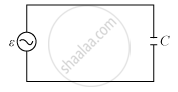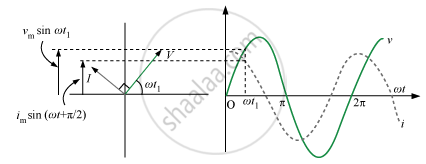Share

# Show that the Current Leads the Voltage in Phase By π/2 in an Ac Circuit Containing an Ideal Capacitor ? - Physics

ConceptAc Voltage Applied to a Capacitor

#### Question

Show that the current leads the voltage in phase by π/2 in an AC circuit containing an ideal capacitor ?

#### Solution

Let us consider a capacitor C connected to an AC source as shown below.Let the AC voltage applied be

v = v_msinωt

∴ v = q/C

Applying Kirchhoff’s loop rule, we have:

 v_msinωt  q/C

i = (dq)/dt

∴ i = (d)/dt( v_mCsinωt)

ωCv_mcos(ωt)

cos ωt = sinωt+π/2

∴ i = i_msin(ωt+π/2)

i_m = ωCv_m

i =(v_m)/((1/(ωC)))Hence, the current leads the voltage in phase by π/2.
Is there an error in this question or solution?

#### APPEARS IN

Solution Show that the Current Leads the Voltage in Phase By π/2 in an Ac Circuit Containing an Ideal Capacitor ? Concept: Ac Voltage Applied to a Capacitor.
S